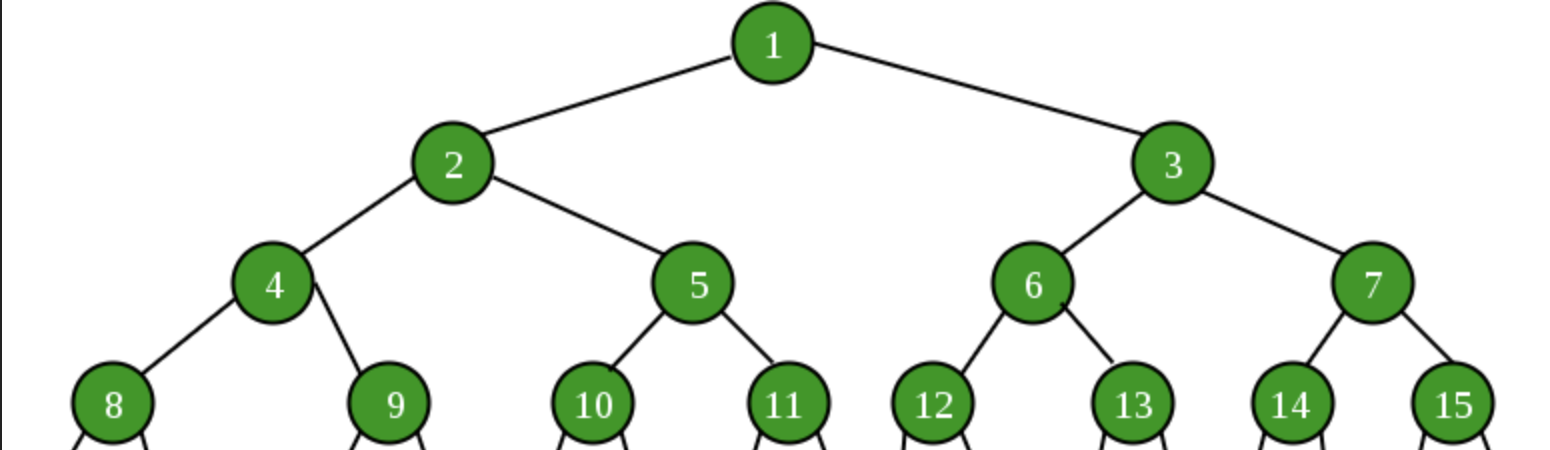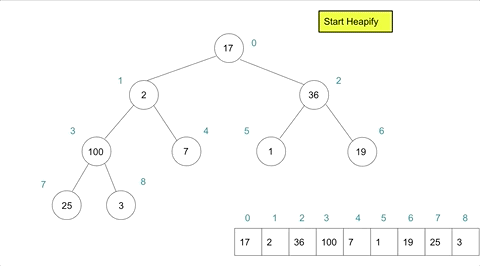<Daniel Sasse/># Algorithm Tutorial: Intro to Heaps - Heapify & Heap Sort

javascript

algorithms

codenewbie

Published: Tue Jun 22 2021 (~ 6 min)

Last week in Algorithm Tutorials, I discussed the Heap data structure, and how it is used to make an optimized data structure for retrieving the max/min value of a series and being able to quickly re-prioritize itself as new values are added in use cases such as a priority queue.

As suggested by @aminmansuri in the comments last week, the amazing properties of a heap do not end here. Let's examine `heapify` and `heapSort`. If you are unfamiliar with the heap structure, and the `bubbleUp` and `trickleDown` manipulations it requires, please first read my previous post

## Contents

### Heapify

Heapify describes the act of taking an existing, unordered array, and transforming it into a Heap structure. What makes this process intriguing, is that if implemented well, it can be done in place, meaning O(1) space, and in linear, O(n), time versus the expected O(n log n) time.

#### Three Approaches

To heapify an existing array, we could take one of three approaches:

1. We create a new empty heap, and then iterate through the array, inserting each element of the array into this heap using the `insert` and `bubbleUp` functions described in the previous entry. The drawback to this approach, is that not only are we creating an entirely new structure to hold each value, O(n) space, we also need to iterate through each item in the array, O(n), and insert each one, O(log n), which creates an overall time complexity of O(n log n). It works, but we can do better.

To improve our space usage, we would need to create the heap by modifying the existing array elements, and shuffling them within this array as needed using the `bubbleUp()` or `trickleDown()` methods.

1. Our first option would be to start at the first item in the array (root of the tree), and iterate through each element and invoking `bubbleUp` on each one. This would compare each node against its ancestors, and move it further up the tree as necessary. By the time we finish with the last element in the array, each element will have been placed accordingly and we will have our heap.
1. The other option, is to start at the bottom-most node on the tree that has children and iterate up to the root, calling `trickleDown` on each one. Like the previous option, this would leave each element correctly place once we finish with the root node.

To compare the efficiency of option 2 and 3 above, we need to closely examine the structure of a heap to see how many potential swaps would need to occur for a given node, and how many nodes could be required to do those swaps.

#### Measuring Efficiency

Let's use a 15 node tree as an example. Mathematically, we can calculate the number of tiers in any tree with `log n` where n is the number of nodes. In this case, that means 4 tiers. Using the approach in option 2, we could find the worst case total number of swaps by looking at the distance from a node's tier to the root.Ex:

• 1 node would have 0 swaps (already the root)
• 2 nodes on tier 2 could have 1 swap to reach the root
• 4 nodes on tier 3 could have 2 swaps to reach the root
• 8 nodes on tier 4 could have 3 swaps to reach the root

Here we can quickly see that as the tree becomes deeper, the number of potential swaps grows quickly since in a tree structure half of the nodes can be in the bottom tier of the tree and will need to potentially swap with the entire depth of the tree. Ultimately, this can be modeled by `n/2 * log n` for any given tier, which simplifies to O(n log n) like option 1, but without the extra needed space.

For comparison, if we used the approach in option 3 and call `trickleDown` on each node, the "swap count" would look very different for our 16 node tree:

Ex:

• 1 node at the root could have 3 swaps to reach the bottom
• 2 nodes on tier 2 could have 2 swaps to reach the bottom
• 4 nodes on tier 3 could have 1 swaps to reach the bottom
• 8 nodes on tier 4 have 0 swaps( already at the bottom)

Here it should be immediately clear that for up to half of the nodes of the tree, no action is necessary, and would thus be more efficient than using option 2 and `bubbleUp`. Mathematically, this process comes out to O(n) time and is supported by this proof provided by Jeremy West. With this process, we can turn any array into a heap without extra space, and in constant time.

#### Heapify Implementation

To efficiently implement heapify, we need to first find the last node in the tree that has children, and call `trickleDown` for each node from there to the root. We can find this node by using `Math.floor((n - 2)/2)`. Unlike in the previous blog, we want the `trickleDown` action to begin at the specified node, and not always at the root, so I have refactored `trickleDown` to accept an optional parameters compared to the implementation in my previous post. See the full MaxHeap class gist below for the `trickleDown` implementation and the rest of the MaxHeap class implementation.

``````class MaxHeap {
constructor(arr = []){
this.values = this._heapify(arr)
}
_heapify(arr){
if (this.size > 0) return // Optional: Prevent overriding existing heap values
this.size = arr.length
/**
* To prevent mutating current array, copy arr with
* this.values = [...arr]
*/
this.values = arr
const nodeCount = this.size - 1
// Finds the last node of the tree that has children
let cIdx = Math.floor((nodeCount - 2)/2)
/** For each node up through the root,
* call trickleDown
*/
for (let i = cIdx; i >= 0; i--){
this._trickleDown(i)
}
return this.values
}
// See gist for rest of class implementation
}``````

If we applied created a heap instance with `arr = [17,2,36,100,7,1,19,25,3]` we could model the `heapify` action as such:### Heap Sort

Heap sort is a sorting method that utilizes the heapify action we built above to sort array using constant space and O(n log n) time. There are essentially two phases to this sorting method:

1. Heapify the array
2. Iterate through the length of the array and for each index put the max value from the heap and place it at the end of the array.

Utilizing what we have already discussed with heapify above, and extraction from the previous post, this action is fairly similar. The major difference is that during extraction we do not want to remove a value from the array with `.pop`, nor do we always want to move the extract value to the last index of the array each time. Instead we can use an index pointer to determine where to place the max value, and where to stop the `trickleDown`

``````  static heapSort(arr){
const heap = new MaxHeap(arr)
for (let i = arr.length - 1; i > 0; i--){
// Place max at pointer position by swapping with root
heap._swap(0,i)
// Begin trickle at root, end before placed value
heap._trickleDown(0, i)
}
return heap.values
}``````

### Resources

These resources below were helpful in putting together this post, and will be helpful if you wish to dig further!